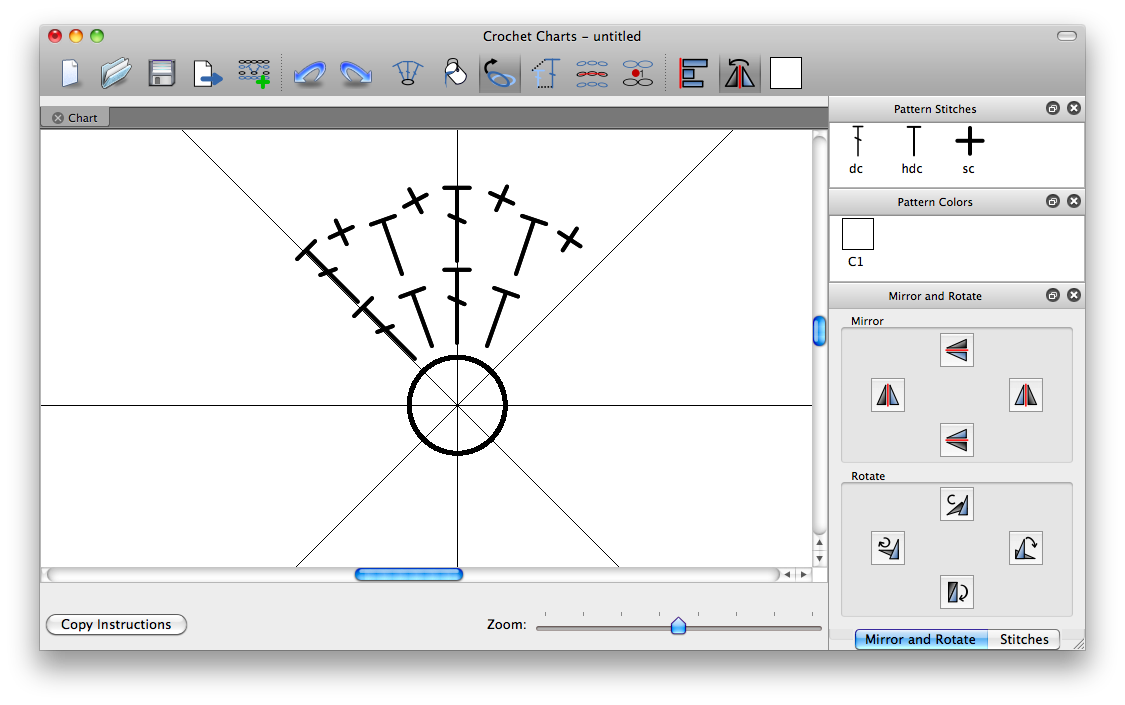## 嵇爾的吐槽

#没事画轮子的嵇尔不定期的(W)碎(E)碎(B)念(B)和(L)吐(O)槽(G)# Crochet ChartsGithub repo 发现了2个，一个人弄的+hash都一样但是居然没看到Fork痕迹：

https://github.com/iPenguin/CrochetCharts

https://github.com/StitchworksSoftware/CrochetCharts

Problem 59 XOR decryption 2017-04-20 09:32:00

Each character on a computer is assigned a unique code and the preferred standard is ASCII (American Standard Code for Information Interchange). For example, uppercase A = 65, asterisk (*) = 42, and lowercase k = 107.

A modern encryption method is to take a text file, convert the bytes to ASCII, then XOR each byte with a given value, taken from a secret key. The advantage with the XOR function is that using the same encryption key on the cipher text, restores the plain text; for example, 65 XOR 42 = 107, then 107 XOR 42 = 65.

For unbreakable encryption, the key is the same length as the plain text message, and the key is made up of random bytes. The user would keep the encrypted message and the encryption key in different locations, and without both “halves”, it is impossible to decrypt the message.

Unfortunately, this method is impractical for most users, so the modified method is to use a password as a key. If the password is shorter than the message, which is likely, the key is repeated cyclically throughout the message. The balance for this method is using a sufficiently long password key for security, but short enough to be memorable.

Your task has been made easy, as the encryption key consists of three lower case characters. Using cipher.txt (right click and ‘Save Link/Target As…’), a file containing the encrypted ASCII codes, and the knowledge that the plain text must contain common English words, decrypt the message and find the sum of the ASCII values in the original text.

Problem 58 Spiral primes 2017-04-18 13:50:00

Starting with 1 and spiralling anticlockwise in the following way, a square spiral with side length 7 is formed.

37 36 35 34 33 32 31
38 17 16 15 14 13 30
39 18 5 4 3 12 29
40 19 6 1 2 11 28
41 20 7 8 9 10 27
42 21 22 23 24 25 26
43 44 45 46 47 48 49

It is interesting to note that the odd squares lie along the bottom right diagonal, but what is more interesting is that 8 out of the 13 numbers lying along both diagonals are prime; that is, a ratio of 8/13 ≈ 62%.

If one complete new layer is wrapped around the spiral above, a square spiral with side length 9 will be formed. If this process is continued, what is the side length of the square spiral for which the ratio of primes along both diagonals first falls below 10%?

It is possible to show that the square root of two can be expressed as an infinite continued fraction.

√ 2 = 1 + 1/(2 + 1/(2 + 1/(2 + … ))) = 1.414213…

By expanding this for the first four iterations, we get:

1 + 1/2 = 3/2 = 1.5 1 + 1/(2 + 1/2) = 7/5 = 1.4 1 + 1/(2 + 1/(2 + 1/2)) = 17/12 = 1.41666… 1 + 1/(2 + 1/(2 + 1/(2 + 1/2))) = 41/29 = 1.41379…

The next three expansions are 99/70, 239/169, and 577/408, but the eighth expansion, 1393/985, is the first example where the number of digits in the numerator exceeds the number of digits in the denominator.

In the first one-thousand expansions, how many fractions contain a numerator with more digits than denominator?

RSS